# How to find distinct values of multiple columns in PySpark ?

• Last Updated : 04 Jul, 2021

In this article, we will discuss how to find distinct values of multiple columns in PySpark dataframe.

Let’s create a sample dataframe for demonstration:

## Python3

 `# importing module``import` `pyspark`` ` `# importing sparksession from pyspark.sql module``from` `pyspark.sql ``import` `SparkSession`` ` `# creating sparksession and giving an app name``spark ``=` `SparkSession.builder.appName(``'sparkdf'``).getOrCreate()`` ` `# list  of employee data``data ``=` `[[``"1"``, ``"Tezas"``, ``"Google"``],``        ``[``"2"``, ``"Mohit Rawat"``, ``"Rakuten"``],``        ``[``"3"``, ``"rohith"``, ``"Geeksforgeeks"``],``        ``[``"4"``, ``"Nancy"``, ``"IBM"``],``        ``[``"1"``, ``"Raghav"``, ``"Wipro"``],``        ``[``"4"``, ``"Komal"``, ``"Amazon"``]]`` ` `# specify column names``columns ``=` `[``'ID'``, ``'NAME'``, ``'Company'``]`` ` `# creating a dataframe from the lists of data``dataframe ``=` `spark.createDataFrame(data, columns)`` ` `dataframe.show()`

Output: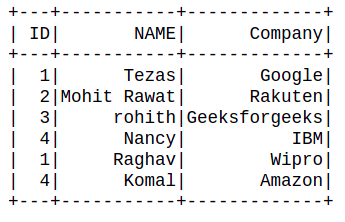Method 1: Using distinct() method

The distinct() method is utilized to drop/remove the duplicate elements from the DataFrame.

Syntax: df.distinct(column)

Example 1: Get a distinct Row of all Dataframe.

## Python3

 `dataframe.distinct().show()`

Output: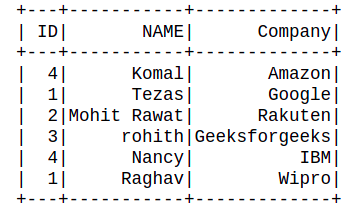Example 2: Get distinct Value of single Columns.

It can be done by passing a single column name with dataframe.

## Python3

 `dataframe.select(``'NAME'``).distinct().show()`

Output: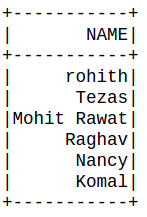Example 3: Get distinct Value of Multiple Columns.

It can be done by passing multiple column names as a form of a list with dataframe.

## Python3

 `dataframe.select(``'ID'``,``"NAME"``).distinct().show()`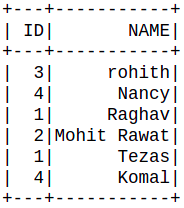Method 2: Using dropDuplicates() method.

The dropDuplicates() used to remove rows that have the same values on multiple selected columns.

Syntax: df.dropDuplicates()

Example 1: Get a distinct Row of all Dataframe.

## Python3

 `dataframe.dropDuplicates().show()`

Output:Example 2: Get distinct Value of single Columns.

It can be done by passing a single column name with dataframe.

## Python3

 `dataframe.select(``"NAME"``).dropDuplicates().show()`

Output:Example 3: Get distinct Value of multiple Columns.

It can be done by passing multiple column names as a form of a list with dataframe.

## Python3

 `dataframe.dropDuplicates([``"NAME"``,``"ID"``]).select([``"ID"``,``"NAME"``]).show()`

Output: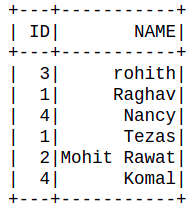My Personal Notes arrow_drop_up